Courses

# Pressure Distribution In A Fluid - 1

## 15 Questions MCQ Test Fluid Mechanics - Notes, Videos, MCQs & PPTs | Pressure Distribution In A Fluid - 1

Description
This mock test of Pressure Distribution In A Fluid - 1 for Civil Engineering (CE) helps you for every Civil Engineering (CE) entrance exam. This contains 15 Multiple Choice Questions for Civil Engineering (CE) Pressure Distribution In A Fluid - 1 (mcq) to study with solutions a complete question bank. The solved questions answers in this Pressure Distribution In A Fluid - 1 quiz give you a good mix of easy questions and tough questions. Civil Engineering (CE) students definitely take this Pressure Distribution In A Fluid - 1 exercise for a better result in the exam. You can find other Pressure Distribution In A Fluid - 1 extra questions, long questions & short questions for Civil Engineering (CE) on EduRev as well by searching above.
QUESTION: 1

### Which one of the following is the unit of pressure?

Solution:

Explanation: Pressure is defined as the force per unit area acting normal to a surface. The SI unit of force is N and area is m2. Thus, the unit of pressure will be N / m2.

QUESTION: 2

### Which one of the following statements is true regarding pressure?

Solution:

Explanation:Pressure is defined as the force per unit area acting normal to a surface. Both force and area are vectors. but the division of one by the other leads to a scalar quantity.

QUESTION: 3

### If the pressure at apoint is 1m of water, what will be it's value in terms of 'm' in oil?(Take the specific gravity of oil to be 0.8)

Solution:
• Explanation: Pressure at a pointy P is equal to ρgh, where ρ is  the density and h is the height of the liquid column. Therefore, ρwater*1*g = ρoil*h*g, where h is the pressure in terms of m of oil.

Thus, h= ρwateroil  = 1/0.8 = 1.25.

QUESTION: 4

A beaker half-filled with water is exposed to the atmosphere. If the pressure at points A, B and C as shown are Pa, Pb and Pc respectively, which one of the following will be the relation connecting the three?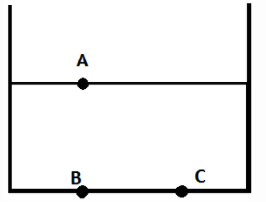Solution:

Explanation: Since the beaker is exposed to the atmosphere, the pressure at point A will be atmospheric, Pa = 0. Pressure increases in the vertically downward direction, Pa < Pb and Pa < Pc.
Pressure remains constant in the horizontal direction, Pb = Pc. Therefore, Pa < Pb = Pc.

QUESTION: 5

A beaker is filled with a liquid up to a height h. If A and B are two points, one on the free surface and one at the base as shown, such that the minimum distance between the two is l, what will be the pressure at point B?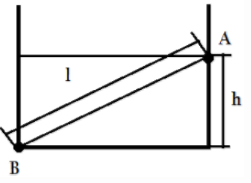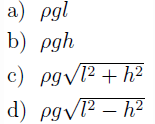Solution:

Explanation: For a constant density liquid, pressure varies linearly in the vertically downward direction. Thus,
PB = PA + ρgh
where PB=Pressure at B, PA=Pressure at A, ρ=density of the liquid, g=acceleration due to gravity and h=vertical distance sePArating the two points. Since A is at the free surface, PA= 0, PB = ρgh.

QUESTION: 6

A beaker of height h is filled with a liquid of density ρ up to a certain limit. The beaker is rotated by an angle θ such that further increase in the angle will result in over flow of the liquid. If the liquid surface is exposed to the atmosphere, what will be the gauge pressure at point B?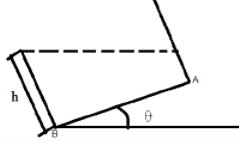Solution:

Explanation: Vertical distance below the free surface at which the point B is located will be h cos θ.
Since the pressure at the free surface is atmospheric, the gauge pressure at B will be = 0 + ρgh cos θ.

QUESTION: 7

An arm of a teapot is completely filled with tea (density=ρ) If the arm has a length of l and is inclined at 30o to the horizontal, what will be the pressure difference between the two points, C at the mouth and D at the base of the arm?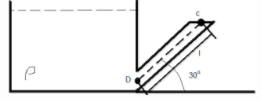Solution:

Explanation: Vertical distance difference between the two points, C at the mouth and D at the base of the arm will be l sin θ = l sin 30o = l=2. Thus, pressure difference between C and D is = ρgl/2.

QUESTION: 8

A beaker is filled with a liquid of density ρ1 up to a certain height. The pressure at the base of the beaker id Pb. If the liquid is replaced by an equal volume of another liquid of density ρ2, what will be the pressure at the base of the beaker now?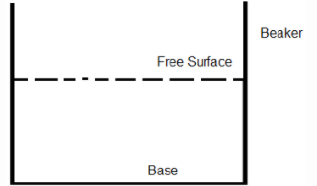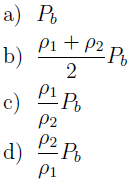Solution:

Explanation: PB = ρ1gh, where h=height up to which the liquid is filled. Since equal volume of the second liquid is poured, it’ll also rise to a height of h. Thus, the pressure at the base will become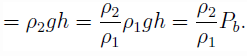QUESTION: 9

A beaker is filled with a liquid of density ρ1 up to a certain height. A is a point, h m downwards from the free surface of the liquid as shown. The liquid is replaced by equal volume of another liquid of density ρ2. If ρ1 > ρ2, how will the pressure at point A change?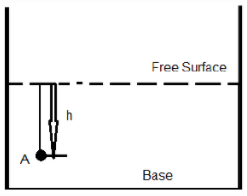Solution:

Explanation: P1= ρ1gh and P2 = ρ2gh, where P1 and P2 are the pressures at point A when liquids of density ρ1 and ρ2 are poured. If ρ1 > ρ2, P1 > P2. Thus the pressure at point A will decrease.

QUESTION: 10

In Figure below, both fluids are at 20°C. If surface tension effects are negligible, what is the density of the oil, in kg/m3?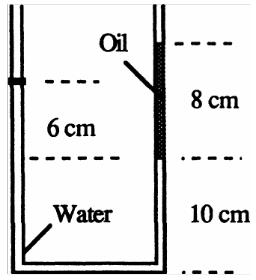Solution:

Explanation: Move around the U-tube from left atmosphere to right atmosphere:

Pa= (9790N/m3)(0.06m) - γoil(0.08m)= Pa

solve for γoil≈ 7343N/m3,

or: ρoil= 7343/9,81≈ 748kg/m3

QUESTION: 11

A beaker of height 15 cm is completely filled with water. Now two-third of the liquid is taken out and an equal amount of two other immiscible liquids of specific gravities 0.8 and 1.2 are poured into the tank. What will be the pressure (in kPa) at a point situated at a height, half the height of the beaker?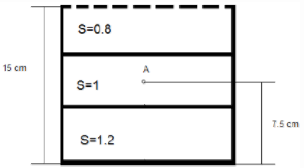Solution:

Explanation: PA = 0.8 * 103 * 9.81 * 0.05 + 1 * 103 * 9.81 * 0.025 = 637.65 kPa.

QUESTION: 12

A beaker is filled with a liquid of density ρ up to a height h. If half the liquid is replaced by equal volume of another liquid of twice the density, what will be the change in the base pressure?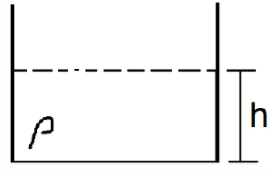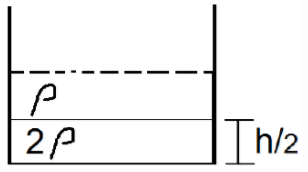Solution:

Explanation: Base pressure when the beaker is filled with a liquid of density ρ up to a height h = ρgh
Base pressure when half the liquid is replaced by equal volume of another liquid of twice the density
= ρg h2 + 2ρg h2 = 32 ρgh
Thus the change in base pressure is = ρgh / 2. Since, P2 > P1, there will be an increase in pressure.

QUESTION: 13

A cuboidal container (each side of 30 cm0) is completely filled with water. A is a point, 25 cm above the base such that the pressure at point A is P. At what height (in cm) from the base will the pressure be 2P?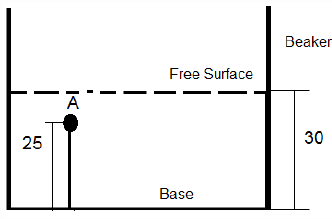Solution:

Explanation: Pressure at a point P is equal to ρgh, where ρ is the density and h is the height of the liquid column from the top. Thus, ρ * g *(30 – h) = 2 * ρ * g *( 30-25), where h from the base where the pressure will be 2P. Thus, h = 30 – 2(30 – 25) = 20.

QUESTION: 14

A closed tank (of height 5 m) is PArtially filled with a liquid as shown. If the pressure of the air above the fluid is 2 bar, find the pressure at the bottom of the tank. Assume the density of the liquid to vary according to the following relation: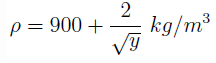where y is the height from the base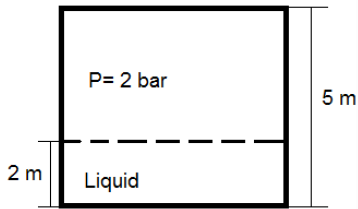Solution:

Explanation: The change of pressure P with vertical direction y is given by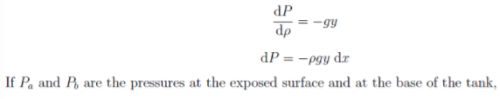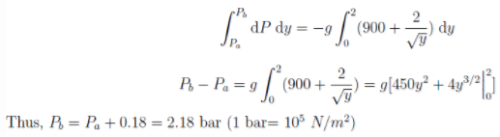QUESTION: 15

The pressure gauges 1, 2 and 3 are installed on the system as shown. If the readings of the gauges be P1 = 1 bar, P2 = 2bar and P3 = 3 bar, what will be the value of P? (Take Patm = 1.01 bar)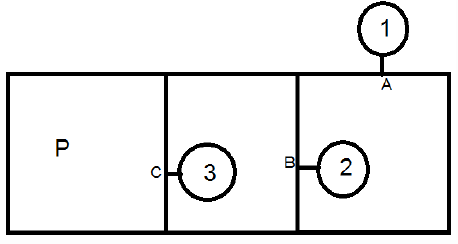Solution:

Explanation: PA = PAtm + P1
PB = PA + P2
PC = PB + P3
P = PC = PAtm + P1 + P2 + P3 = 1.01 + 1 + 2 + 3 = 7.01.